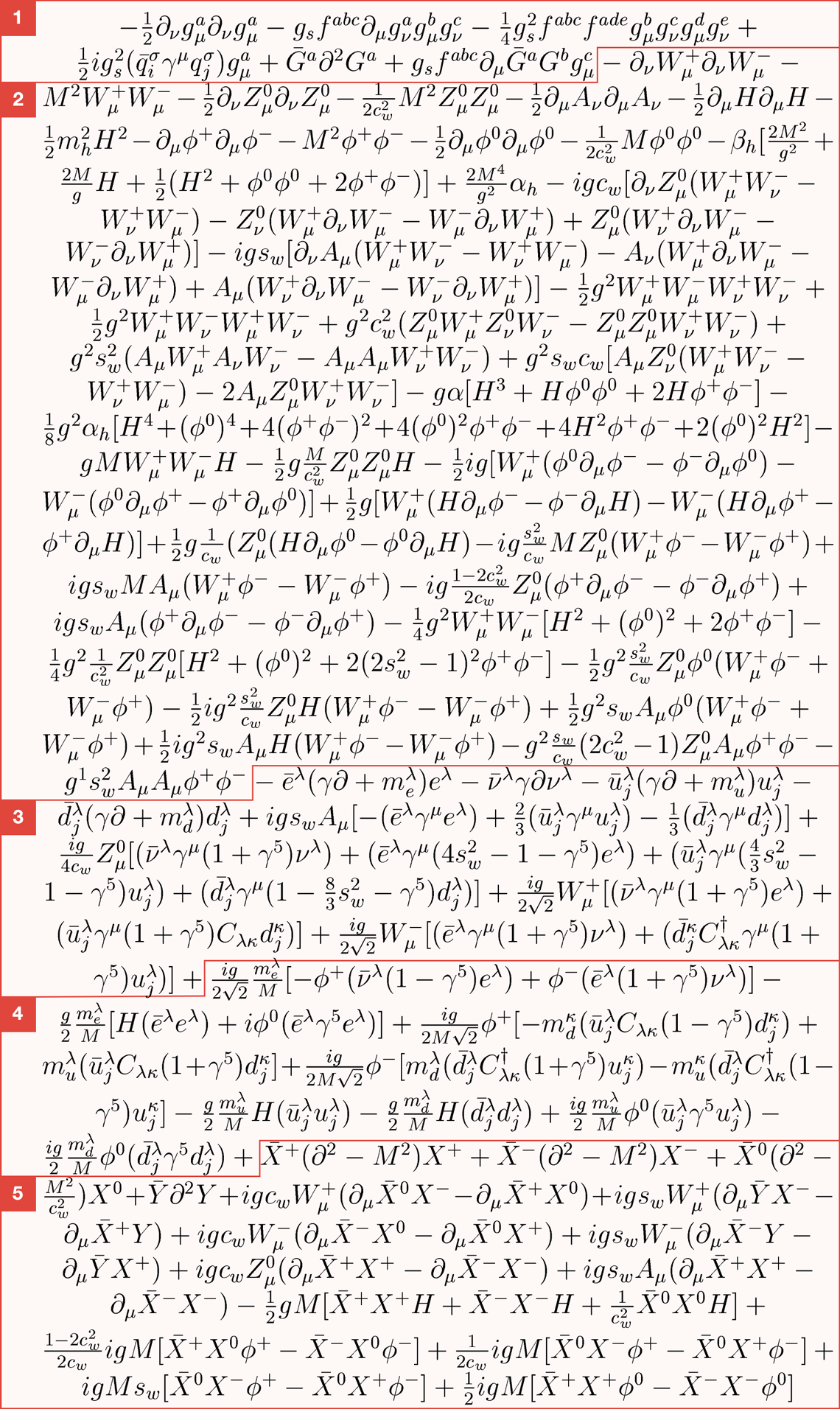# Standard Model of particle physics written in the LagrangianSection 1
These three lines in the Standard Model are ultraspecific to the gluon, the boson that carries the strong force. Gluons come in eight types, interact among themselves and have what’s called a color charge.

Section 2
Almost half of this equation is dedicated to explaining interactions between bosons, particularly W and Z bosons.
Bosons are force-carrying particles, and there are four species of bosons that interact with other particles using three fundamental forces. Photons carry electromagnetism, gluons carry the strong force and W and Z bosons carry the weak force. The most recently discovered boson, the Higgs boson, is a bit different; its interactions appear in the next part of the equation.

Section 3
This part of the equation describes how elementary matter particles interact with the weak force. According to this formulation, matter particles come in three generations, each with different masses. The weak force helps massive matter particles decay into less massive matter particles.
This section also includes basic interactions with the Higgs field, from which some elementary particles receive their mass.
Intriguingly, this part of the equation makes an assumption that contradicts discoveries made by physicists in recent years. It incorrectly assumes that particles called neutrinos have no mass.

Section 4
In quantum mechanics, there is no single path or trajectory a particle can take, which means that sometimes redundancies appear in this type of mathematical formulation. To clean up these redundancies, theorists use virtual particles they call ghosts.
This part of the equation describes how matter particles interact with Higgs ghosts, virtual artifacts from the Higgs field.

Section 5
This last part of the equation includes more ghosts. These ones are called Faddeev-Popov ghosts, and they cancel out redundancies that occur in interactions through the weak force.

Thomas Gutierrez, an assistant professor of Physics at California Polytechnic State University, transcribed the Standard Model Lagrangian for the web. He derived it from Diagrammatica, a theoretical physics reference written by Nobel Laureate Martinus Veltman. In Gutierrez’s dissemination of the transcript, he noted a sign error he made somewhere in the equation.

in Symmetry